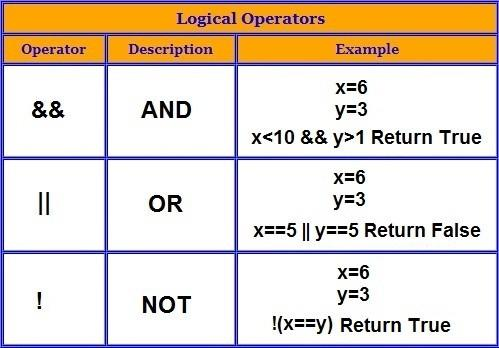# Logical operator

## Introduction

Logic NOT

Logic AND

Logic OR

The priority is: NOT AND OR

p>

Same level operations from left to right

In formal logic, logical operators or logical connectives connect sentences into more Complicated complex sentences. For example, suppose there are two logical propositions, "It's raining" and "I'm in the house", we can compose them into a complex proposition "It's raining, and I'm in the house" or "No is raining" or "If it is raining, then I am in the house". A new sentence or proposition composed of two sentences is called compound sentence or compound proposition.

Table 15-7. Logical operators

Example

th>

Name

Result

\$a and \$b

And (logical AND)

TRUE, if both \$a and \$b are TRUE.

\$a or \$b

Or (logical OR)

< /td>

TRUE, if either \$a or \$b is TRUE.

\$a xor \$b

Xor (logical exclusive OR)

TRUE, if \$a or \$b are different.

! \$a

Not (logical not)

TRUE, if \$a is not TRUE.

\$a && \$b

And (logical AND) p>

TRUE, if both \$a and \$b are TRUE.

\$a || \$b

Or (logical OR)

TRUE, if either \$a or \$b is TRUE.

The reason why there are two different operators of "and" and "or" is that they have different operating precedences (see Operator Precedence).

## Basic operators

Basic operators are: "not" (¬), "and" (∧), "or" (∨), "condition" (→) And "double conditions" (↔). "Not" is a unary operator, it only operates one item (¬ P). The rest is a binary operator, which operates two items to form a complex statement (P ∧ Q, P ∨ Q, P → Q, P ↔ Q).

Pay attention to the similarity of the symbol "and" (∧) and intersection (∩), "or" (∨) and union (∪). This is no coincidence: the definition of intersection uses "and", and the definition of union uses "or."

The truth table of these connectors:

< th>

T

< /tr>

P

th>

Q

¬P

P ∧ and Q

P ∨ or Q< /i>

PQ

PQ

T

T

F

T

T

T

T

F

F

F

T

F

< p>F

F

T

T

F

T

T

F

F

th>

F

T

F

F

T

T

In order to reduce the number of brackets required, there are the following priority rules: ¬ is higher than ∧, ∧ is higher than ∨, and ∨ is higher than →. For example, P ∨ Q ∧ ¬ R → S is a convenient way of writing (P ∨ (Q ∧ (¬ R)) → S.